## Applications of First‐Order Equations

Orthogonal trajectories. The term orthogonal means perpendicular, and trajectory means path or cruve. Orthogonal trajectories, therefore, are two families of curves that always intersect perpendicularly. A pair of intersecting curves will be perpendicular if the product of their slopes is −1, that is, if the slope of one is the negative reciprocal of the slope of the other. Since the slope of a curve is given by the derivative, two familes of curves ƒ 1( x, y, c) = 0 and ƒ 2( x, y, c) = 0 (where c is a parameter) will be orthogonal wherever they intersect ifExample 1: The electrostatic field created by a positive point charge is pictured as a collection of straight lines which radiate away from the charge (Figure ). Using the fact that the equipotentials (surfaces of constant electric potential) are orthogonal the electric field lines, determine the geometry of the equipotenitials of a point charge.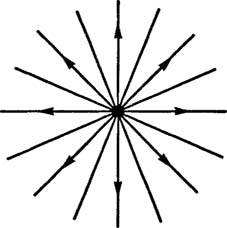Figure 1

If the origin of an xy coordinate system is placed at the charge, then the electric field lines can be described by the family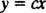The first step in determining the orthogonal trajectories is to obtain an expression for the slope of the curves in this family that does not involve the parameter c. In the present case,The differential equation describing the orthogonal trajectories is thereforesince the right‐hand side of (**) is the negative reciprocal of the right‐hand side of (*). Because this equation is separable, the solution can proceed as follows: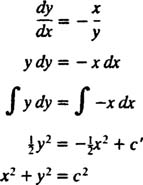where c 2 = 2 c′.

The equipotential lines (that is, the intersection of the equipotential surfaces with any plane containing the charge) are therefore the family of circles x 2 + y 2 = c 2 centered at the origin. The equipotential and electric field lines for a point charge are shown in Figure 2.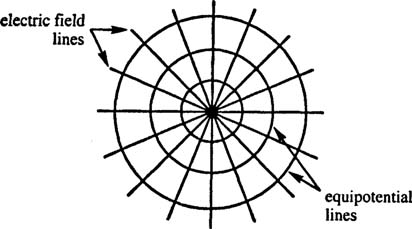Figure 2

Example 2: Determine the orthogonal trajectories of the family of circles x 2 + ( yc) 2 = c 2 tangent to the x axis at the origin.

The first step is to determine an expression for the slope of the curves in this family that does not involve the parameter c. By implicit differentiation,To eliminate c, note thatThe expression for dy/dx may now be written in the formTherefore, the differential equation describing the orthogonal trajectories issince the right‐hand side of (**) is the negative reciprocal of the right‐hand side of (*).

If equation (**) is written in the form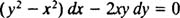note that it is not exact (since M y = 2 y but N x = −2 y). However, because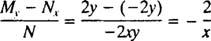is a function of x alone, the differential equation hasas an integrating factor. After multiplying through by μ = x −2, the differential equation describing the desired family of orthogonal trajectories becomes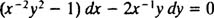which is now exact (because M y = 2 x −2 y = N x ). Since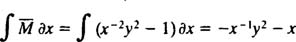andthe solution of the differential equation is(The reason the constant was written as −2 c rather than as c will be apparent in the following calculation.) With a little algebra, the equation for this family may be rewritten:This shows that the orthogonal trajectories of the circles tangent to the x axis at the origin are the circles tangent to the y axis at the origin! See Figure 3.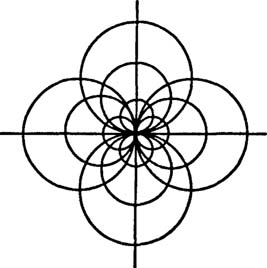Figure 3

Radioactive decay. Some nuclei are energetically unstable and can spontaneously transform into more stable forms by various processes known collectively as radioactive decay. The rate at which a particular radioactive sample will decay depends on the identity of the sample. Tables have been compiled which list the half‐lives of various radioisotopes. The half‐life is the amount of time required for one‐half the nuclei in a sample of the isotope to decay; therefore, the shorter the half‐life, the more rapid the decay rate.

The rate at which a sample decays is proportional to the amount of the sample present. Therefore, if x(t) denotes the amount of a radioactive substance present at time t, then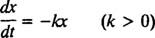(The rate dx/ dt is negative, since x is decreasing.) The positive constant k is called the rate constant for the particular radioisotope. The solution of this separable first‐order equation iswhere x o denotes the amount of substance present at time t = 0. The graph of this equation (Figure 4) is known as the exponential decay curve:Figure 4

The relationship between the half‐life (denoted T 1/2) and the rate constant k can easily be found. Since, by definition, x = ½ x 6 at t = T 1/2, (*) becomes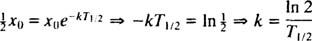Because the half‐life and rate constant are inversely proportional, the shorter the half‐life, the greater the rate constant, and, consequently, the more rapid the decay.

Radiocarbon dating is a process used by anthropologists and archaeologists to estimate the age of organic matter (such as wood or bone). The vast majority of carbon on earth is nonradioactive carbon‐12 ( 12C). However, cosmic rays cause the formation of carbon‐14 ( 14C), a radioactive isotope of carbon which becomes incorporated into living plants (and therefore into animals) through the intake of radioactive carbon dioxide ( 14CO 2). When the plant or animal dies, it ceases its intake of carbon‐14, and the amount present at the time of death begins to decrease (since the 14C decays and is not replenished). Since the half‐life of 14C is known to be 5730 years, by measuring the concentration of 14C in a sample, its age can be determined.

Example 3: A fragment of bone is discovered to contain 20% of the usual 14C concentration. Estimate the age of the bone.

The relative amount of 14C in the bone has decreased to 20% of its original value (that is, the value when the animal was alive). Thus, the problem is to calculate the value of t at which x( t) = 0.20 x o (where x = the amount of 14C present). Sincethe exponential decay equation (*) says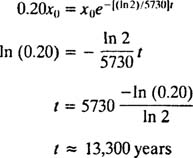Newton's Law of Cooling. When a hot object is placed in a cool room, the object dissipates heat to the surroundings, and its temperature decreases. Newton's Law of Cooling states that the rate at which the object's temperature decreases is proportional to the difference between the temperature of the object and the ambient temperature. At the beginning of the colling process, the difference between these temperatures is greatest, so this is when the rate of temperature decrease is greatest. However, as the object cools, the temperature difference gets smaller, and the cooling rate decreases; thus, the object cools more and more slowly as time passes. To formulate this process mathematically, let T( t) denote the temperature of the object at time t and let T s denote the (essentially constant) temperature of the surroundings. Newton's Law of Cooling then saysSince T s < T (that is, since the room is cooler than the object), T decreases, so the rate of change of its temperature, dT/dt, is necessarily negative. The solution of this separable differential equation proceeds as follows:Example 4: A cup of coffee (temperature = 190°F) is placed in a room whose temperature is 70°F. After five minutes, the temperature of the coffee has dropped to 160°F. How many more minutes must elapse before the temperature of the coffee is 130°F?

Assuming that the coffee obeys Newton's Law of Cooling, its temperature T as a function of time is given by equation (*) with T s = 70:Because T(0) = 190, the value of the constant of integration ( c) can be evaluated:Furthermore, since information about the cooling rate is provided ( T = 160 at time t = 5 minutes), the cooling constant k can be determined: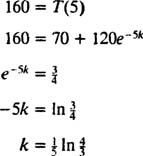Therefore, the temperature of the coffee t minutes after it is placed in the room is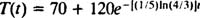Now, setting T = 130 and solving for t yieldsThis is the total amount of time after the coffee is initially placed in the room for its temperature to drop to 130°F. Therefore, after waiting five minutes for the coffee to cool from 190°F to 160°F, it is necessary to then wait an additional seven minutes for it to cool down to 130°F.

Skydiving. Once a sky diver jumps from an airplane, there are two forces that determine her motion: the pull of the earth's gravity and the opposing force of air resistance. At high speeds, the strength of the air resistance force (the drag force) can be expressed as kv 2, where v is the speed with which the sky diver descends and k is a proportionality constant determined by such factors as the diver's cross‐sectional area and the viscosity of the air. Once the parachute opens, the descent speed decreases greatly, and the strength of the air resistance force is given by Kv.

Newton's Second Law states that if a net force F net acts on an object of mass m, the object will experience an accelaration a given by the simple equation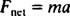Since the acceleration is the time derivative of the velocity, this law can be expressed in the formIn the case of a sky diver initially falling without a parachute, the drag force is F drag = kv 2, and the equation of motion (*) becomes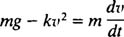or more simply,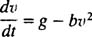where b = k/m. [The letter g denotes the value of the gravitational acceleration, and mg is the force due to gravity acting on the mass m (that is, mg is its weight). Near the surface of the earth, g is approximately 9.8 meters per second 2.] Once the sky diver's descent speed reaches

vthe preceding equation says dv/ dt = 0; that is, v stays constant. This occurs when the speed is great enough for the force of air resistance to balance the weight of the sky diver; the net force and (consequetly) the acceleration drop to zero. This constant descent velocity is known as the terminal velocity. For a sky diver falling in the spread‐eagle position without a parachute, the value of the proportionality constant k in the drag equation F drag = kv 2 is approximately ¼ kg/m. Therefore, if the sky diver has a total mass of 70kg (which corresponds to a weight of about 150 pounds), her terminal velocity is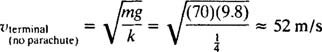or approximately 120 miles per hour.

Once the parachute opens, the air resistance force becomes F air resist = Kv, and the equation of motion (*) becomes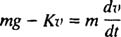or more simply,where B = K/m. Once the parachutist's descent speed slows to v = g/B = mg/K, the preceding equation says dv/dt = 0; that is, v stays constant. This occurs when the speed is low enough for the weight of the sky diver to balance the force of air resistance; the net force and (consequently) the acceleration reach zero. Again, this constant descent velocity is known as the terminal velocity. For a sky diver falling with a parachute, the value of the proportionality constant K in the equation F air resist = Kv is approximately 110 kg/s. Therefore, if the sky diver has a total mass of 70 kg, the terminal velocity (with the parachute open) is only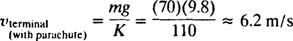which is about 14 miles per hour. Since it is safer to hit the ground while falling at a rate of 14 miles per hour rather than at 120 miles per hour, sky divers use parachutes.

Example 5: After a free‐falling sky diver of mass m reaches a constant velocity of v 1, her parachute opens, and the resulting air resistance force has strength Kv. Derive an equation for the speed of the sky diver t seconds after the parachute opens.

Once the parachute opens, the equation of motion is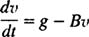where B = K/m. The parameter that will arise from the solution of this first‐order differential equation will be determined by the initial condition v(0) = v 1 (since the sky diver's velocity is v 1 at the moment the parachute opens, and the “clock” is reset to t = 0 at this instant). This separable equation is solved as follows:Now, since v(0) = v 1gBv 1 = c, the desired equation for the sky diver's speed t seconds after the parachute opens isNote that as time passes (that is, as t increases), the term e −( K/m)t goes to zero, so (as expected) the parachutist's speed v slows to mg/K, which is the terminal speed with the parachute open.